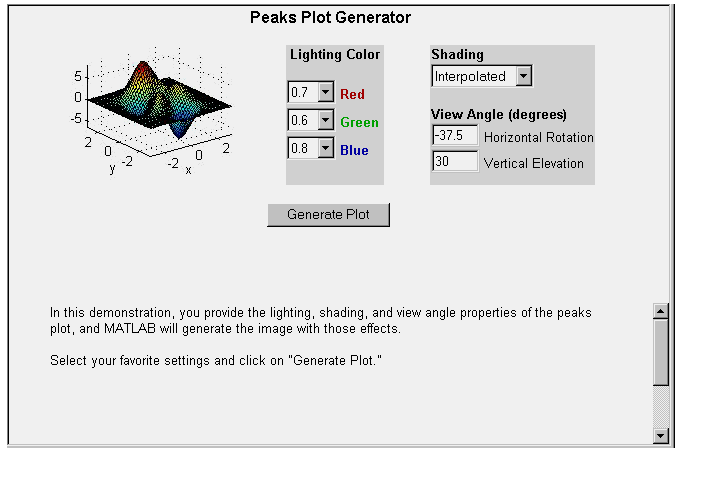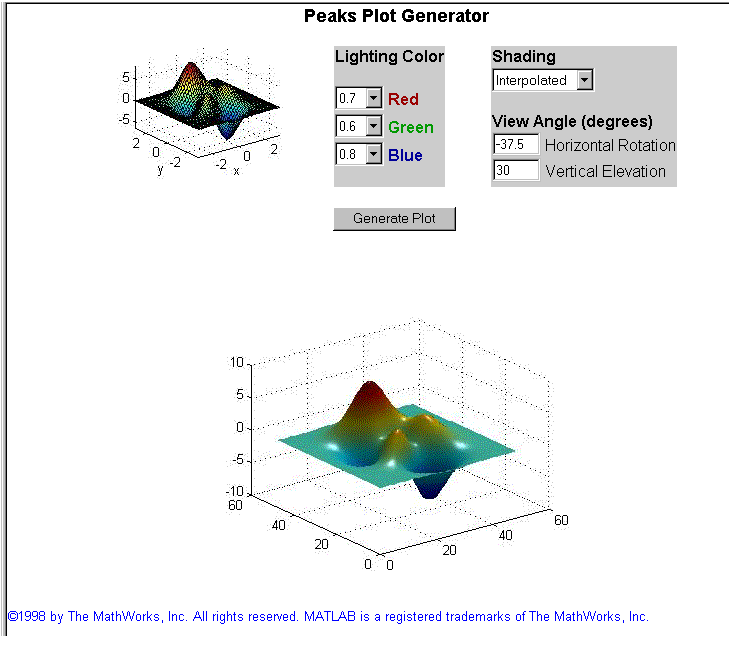MATLAB Web ServerMATLAB Graphics

The `webpeaks` function, included as a demonstration program, creates a `peaks` plot and returns the output to your Web browser. In examining portions of the `webpeaks` code, you will see how to include MATLAB graphics as part of a MATLAB Web Server application.

To start the `webpeaks` demonstration, set the URL in your browser to
`http://<your_domain>/webpeaks1.html`, the `webpeaks` input document.This input document allows you to set the characteristics of the `peaks` plot you want to generate. This is a more complex input document than the one we used with `webmagic`, as it makes use of HTML frames. The code in the source file

• ```<form action="/cgi-bin/matweb.exe" method="POST"
target="outputwindow">
<input type="hidden" name="mlmfile" value="webpeaks">
```

calls the `webpeaks` function and targets the output to a frame on the lower portion of the input document itself.

In addition to the code necessary to compute and display the `peaks` function, the file `webpeaks.m` contains additional code specific to the transmission of graphics data across the Web. In `webpeaks.m` the code

• ```mlid = getfield(h,'mlid')
```

extracts `mlid` from the structure `h`.

`mlid` is a unique identifier that `matlabserver` provides. Using the value of `mlid` to construct filenames ensures that filenames are unique. It can also be used to maintain contexts among the different connections in an application.You can see this in the code

• ```s.GraphFileName = sprintf('%speaks.jpeg',mlid)
```

which creates a name for a `jpeg` file. If `mlid` has the value `ml00277`, for example, the `jpeg` file will be named `ml00277peaks.jpeg`.

The function `htmlrep` replaces MATLAB variable names it finds in the HTML output template file `webpeaks2.html` with the values in the input structure `s`.

• ```rs = `htmlrep`(s,'webpeaks2.html')
```

`\$GraphFileName\$`, the variable that represents the graphic output, is found in the line

• ```<img border=0 src="\$GraphFileName\$">
```

in `webpeaks2.html`.

The final output document shows both the input and output frames.Note    When the input form contains a "clickable" image created by an `` tag of the form ``, the variable names for the `x` and `y` coordinates are normally passed to the program as `mymap.x` and `mymap.y`. The MATLAB Web Server converts these to `mymap_x` and `mymap_y`. For example, the input ` `results in storing the `x` and `y` coordinates `mymap_x` and `mymap_y` in the structure passed to your program.Additional Application Examples Stock Price Simulation